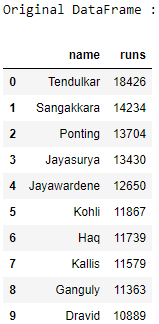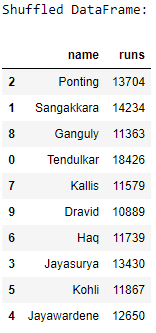Open in App
Not now

# Pandas – How to shuffle a DataFrame rows

• Last Updated : 28 Nov, 2022

Let us see how to shuffle the rows of a DataFrame. We will be using the sample() method of the pandas module to randomly shuffle DataFrame rows in Pandas. Algorithm :

1. Import the pandas and numpy modules.
2. Create a DataFrame.
3. Shuffle the rows of the DataFrame using the sample() method with the parameter frac as 1, it determines what fraction of total instances need to be returned.
4. Print the original and the shuffled DataFrames.

## python

 `# import the modules` `import` `pandas as pd` `import` `numpy as np`   `# create a DataFrame` `ODI_runs ``=` `{``'name'``: [``'Tendulkar'``, ``'Sangakkara'``, ``'Ponting'``,` `                      ``'Jayasurya'``, ``'Jayawardene'``, ``'Kohli'``,` `                      ``'Haq'``, ``'Kallis'``, ``'Ganguly'``, ``'Dravid'``],` `            ``'runs'``: [``18426``, ``14234``, ``13704``, ``13430``, ``12650``,` `                     ``11867``, ``11739``, ``11579``, ``11363``, ``10889``]}` `df ``=` `pd.DataFrame(ODI_runs)`   `# print the original DataFrame` `print``("Original DataFrame :")` `print``(df)`   `# shuffle the DataFrame rows` `df ``=` `df.sample(frac ``=` `1``)`   `# print the shuffled DataFrame` `print``("\nShuffled DataFrame:")` `print``(df)`

Output :My Personal Notes arrow_drop_up
Related Articles